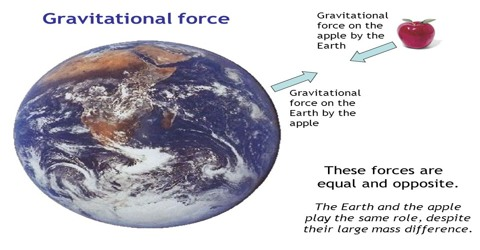# Gravitational Force

Nature of gravitational force:

If a body is released from above and allowed to fall without resistance it falls downward i.e., it falls on the surface of the earth. For example when we thrown a stone upwards from earth it falls down to the earth due to the gravitational force of earth. In the similar way moon is orbiting around earth due to the gravitational force of the earth. Although Gravitational force is the weakest force among the four fundamental forces of nature.

(i) Gravitational force is attractive force between two bodies.

(ii) It acts along the line joining the two bodies.

(iii) Gravitational force does not depend on the medium.

(iv) Gravitational force is proportional to the product of the masses of the two bodies.

Mangoes of a mango tree always fall on the ground. But have you ever thought why the mangoes do not go upward? Actually the earth attracts any objects towards the centre. Again, the body also attracts the earth with equal and opposite force. Compared to anybody on earth, mass of the earth is manyfold heavier. So, motion of the earth toward, the body is negligibly small, hence the object always falls towards the earth, but the earth never moves towards the body.

From the above discussion it is clear that, the earth attracts each body towards its centre. This force of attraction is the weight of the body. That means weight is the applied gravity on the body. The weight always acts directly downward through the centre of gravity of the body.

If the weight of a body of mass m is W, then we can write;

W = mg

i.e., weight = mass x acceleration due to gravity

It the value of acceleration due to gravity changes then weight of the body also changes proportionately. That means, weight of a body is variable, it is not space independent. Weight of a body is not a fundamental property. A body may have weight or may not have.